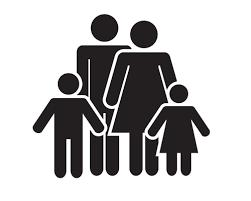# Daughter 6165

His father is 43 years old, and his daughter is 17. How many years ago was a father three times older than a daughter?

x =  4

### Step-by-step explanation:

(43-x)=3·(17-x)

2x = 8

x = 8/2 = 4

x = 4

Our simple equation calculator calculates it.Did you find an error or inaccuracy? Feel free to write us. Thank you!

Tips for related online calculators
Do you have a linear equation or system of equations and looking for its solution? Or do you have a quadratic equation?
Do you want to convert time units like minutes to seconds?